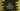# C++ program to convert a hexadecimal value to binary## C++ program to convert a hexadecimal value to binary:

In this post, we will learn how to convert a hexadecimal value to binary in C++. The program will take a hexadecimal value as input from the user, convert it to binary and print that.

### Hexadecimal and binary number system:

Hexadecimal and binary, both are different number systems. The hexadecimal number system uses 16 as the base. For decimal numbers 0 to 9, hexadecimal uses 0 to 9. For 10 to 15, it uses A to F. We can also use a to f. We have to use letters because we need to represent all two digit numbers using one character.

The binary number system uses 2 as base. So, all numbers are represented in 0 and 1 in the binary system.

### Algorithm:

We will use the below algorithm to convert a hexadecimal value to binary:

• Get the hexadecimal value as an input from the user.
• For each character of the hexadecimal value, convert it to binary.
• Join all binary values to get the final binary result.

For example, if we want to convert 2A9B to binary,

• Binary equivalent of 2 is 0010
• Binary equivalent of A is 1010
• Binary equivalent of 9 is 1001
• Binary equivalent of B is 1011

So, the binary of 2A9B is 0010101010011011.

• The program will iterate through the characters of the hexadecimal string one by one.
• It will initialize a result string as an empty string to hold the final binary conversion.
• It will use a loop to iterate through the characters of the string one by one. For each character, it will find the binary equivalent and add it to the result string.

### C++ program:

Below is the complete C++ program:

``````#include <iostream>
#include <string>

using namespace std;

string getBinary(char c)
{
switch (c)
{
case '0':
return "0000";
case '1':
return "0001";

case '2':
return "0010";

case '3':
return "0011";

case '4':
return "0100";

case '5':
return "0101";

case '6':
return "0110";

case '7':
return "0111";

case '8':
return "1000";

case '9':
return "1001";

case 'A':
case 'a':
return "1010";

case 'B':
case 'b':
return "1011";

case 'C':
case 'c':
return "1100";

case 'D':
case 'd':
return "1101";

case 'E':
case 'e':
return "1110";

case 'F':
case 'f':
return "1111";
default:
return "-1";
}
}

int main()
{
string hexString;
string binaryString = "";

cout << "Enter the hexadecimal string: " << endl;
cin >> hexString;

for (int i = 0; i < hexString.length(); i++)
{
binaryString += getBinary(hexString[i]);
}

cout << "Binary: " << binaryString << endl;

return 0;
}``````

Here,

• getBinary is a method to get the binary string for a given character. It takes a character as its parameter and returns the binary equivalent.
• It uses a switch-case block. We have defined the binary equivalent of all possible hexadecimal characters.
• hexString variable is initialized to hold the hexadecimal string and binaryString is to hold the final binary string. binaryString is initialized as an empty string.
• It asks the user to enter the hexadecimal string and reads the string to the hexString variable.
• The for loop iterates through the characters of the hexadecimal string one by one.
• For each character, it finds the binary equivalent by calling the getBinary method.
• It appends the binary string to the end of binaryString.
• At the end of the program, it prints the binary string binaryString to the user.

If you run this program, it will give output as like below:

``````Enter the hexadecimal string:
2a9b
Binary: 0010101010011011``````

It will work for all valid hexadecimal strings. But, it will not show any error message for invalid strings. For example,

``````Enter the hexadecimal string:
2ty
Binary: 0010-1-1``````

This is because, the getBinary method returns -1 for invalid hexadecimal characters and we don’t have any checks for invalid characters.

### Method 2: Handle invalid hexadecimal inputs:

Let’s change the above method a little bit to handle invalid hexadecimal inputs:

``````#include <iostream>
#include <string>

using namespace std;

string getBinary(char c)
{
switch (c)
{
case '0':
return "0000";
case '1':
return "0001";

case '2':
return "0010";

case '3':
return "0011";

case '4':
return "0100";

case '5':
return "0101";

case '6':
return "0110";

case '7':
return "0111";

case '8':
return "1000";

case '9':
return "1001";

case 'A':
case 'a':
return "1010";

case 'B':
case 'b':
return "1011";

case 'C':
case 'c':
return "1100";

case 'D':
case 'd':
return "1101";

case 'E':
case 'e':
return "1110";

case 'F':
case 'f':
return "1111";
default:
return "-1";
}
}

int main()
{
string hexString;
string binaryString = "";

bool isValid = true;
string binary;

cout << "Enter the hexadecimal string: " << endl;
cin >> hexString;

for (int i = 0; i < hexString.length(); i++)
{
binary = getBinary(hexString[i]);
if (binary == "-1")
{
isValid = false;
break;
}
binaryString += binary;
}

if (isValid)
{
cout << "Binary: " << binaryString << endl;
}
else
{
cout << "Invalid hexadecimal input" << endl;
}

return 0;
}``````

Following are the changes I did for this program:

• One boolean value isValid is initialized as true. This variable defines if the input is a valid input or not.
• Another string variable binary is initialized to hold the binary value for a hexadecimal character.
• Inside the for loop, the return value of getBinary is stored in the binary variable first before appending to binaryString. If the value of binary is -1, i.e. if any character in the hexadecimal string is invalid, it assigns false to isValid and exits from the loop.
• After the for loop is completed, it checks the value of isValid and prints a message based on that.

It will give outputs as like below:

``````Enter the hexadecimal string:
2ae7
Binary: 0010101011100111